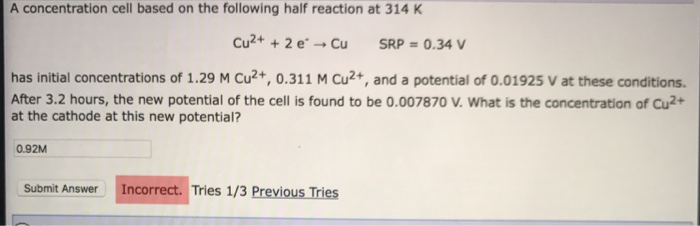# Question & Answer: A concentration cell based on the following half reaction at 314 K Cu^2+ + 2 e^-rightarrow Cu…..A concentration cell based on the following half reaction at 314 K Cu^2+ + 2 e^-rightarrow Cu SRP = 0.34 V has initial concentrations of 1.29 M Cu^2+, 0.311 M Cu^2+, and a potential of 0.01925 V at these conditions After 3.2 hours, the new potential of the cell is found to be 0.007870 V. What is the concentration of Cu^2+ at the cathode at this new potential?

When the cell is NOT under standard conditions, i.e. 1M of each reactants at T = 25°C and P = 1 atm; then we must use Nernst Equation.

The equation relates E°cell, number of electrons transferred, charge of 1 mol of electron to Faraday and finally, the Quotient retio between products/reactants

Don't use plagiarized sources. Get Your Custom Essay on
Question & Answer: A concentration cell based on the following half reaction at 314 K Cu^2+ + 2 e^-rightarrow Cu…..
GET AN ESSAY WRITTEN FOR YOU FROM AS LOW AS \$13/PAGE

The Nernst Equation:

Ecell = E0cell – (RT/nF) x lnQ

In which:

Ecell = non-standard value

E° or E0cell or E°cell or EMF = Standard EMF: standard cell potential
R is the gas constant (8.3145 J/mol-K)
T is the absolute temperature = 298 K
n is the number of moles of electrons transferred by the cell’s reaction
F is Faraday’s constant = 96485.337 C/mol or typically 96500 C/mol
Q is the reaction quotient, where

Q = [C]^c * [D]^d / [A]^a*[B]^b

pure solids and pure liquids are not included. Also note that if we use partial pressure (for gases)

Q = P-A^a / (P-B)^b

substitute in Nernst Equation:

Ecell = E° – (RT/nF) x lnQ

Q = [Cu2+]ox / [Cu2+]red

note that the oxidized Cu2+ will increase, the reduced Cu2+ must decrease

Eº = 0.34 – 0.34 = 0 V at std conditions, therefore, only concentration drives this reaction

so

Ecell = Eº – 8.314*314/(2*96500) * ln ( [Cu2+]ox / [Cu2+]red)

initially:

Ecell = 0 – 8.314*314/(2*96500) * ln (0.311/ 1.29) = 0.019242 V which is the same as given before…

[Cu2+]ox = 0.311 + x

[Cu2+]red = 1.29 – x

E cell goes to 0.00787

0.00787 = 0 – 8.314*314/(2*96500) * ln ( (0.311 + x) /( 1.29 – x))

solve for x…

0.00787 = 0 – 8.314*314/(2*96500) * ln ( (0.311 + x) /( 1.29 – x))

0.00787/(-0.013526) =  ln ( (0.311 + x) /( 1.29 – x))

-0.581842 =  ln ( (0.311 + x) /( 1.29 – x))

exp(-0.581842) = (0.311 + x) /( 1.29 – x)

0.55886 * 1.29 – 0.55886x = 0.311 + x

(1+0.55886)x = 0.55886*1.29 – 0.311

x = 0.4099/1.55886 = 0.26294

substitute:

in the cathode = reduction so

[Cu2+]red = 1.29 – x = 1.29 – 0.26294 = 1.02706 M of Cu2+ left in cathode